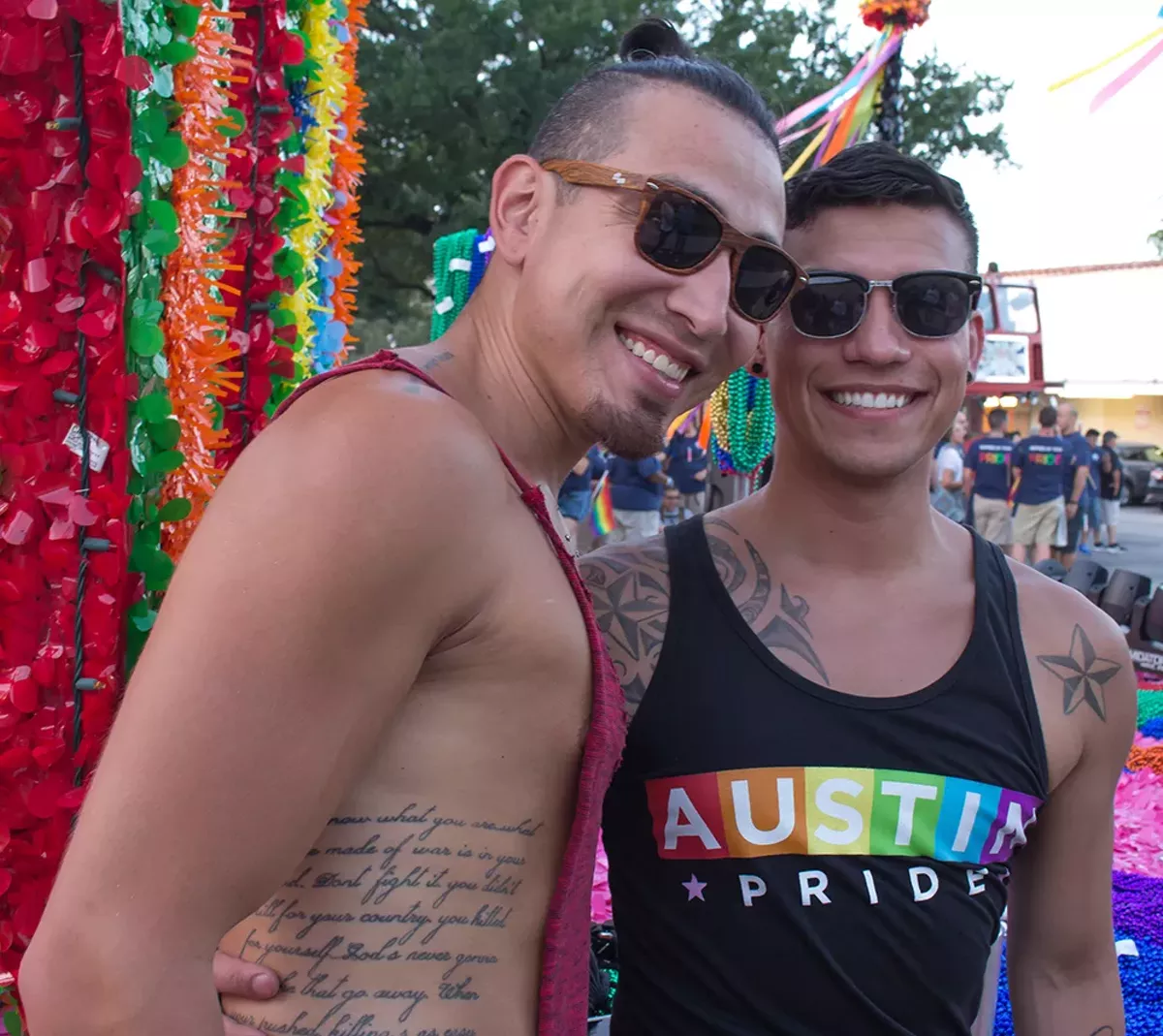# More of Our Favorite Moments from Pride 2017

Share on Facebook
Tweet
Submit to Reddit
Email
Photos by Julian P. Ledezma
OF 93
PREV NEXTJulian P. Ledezma
Julian P. Ledezma
Julian P. Ledezma
Julian P. Ledezma
Julian P. Ledezma
Julian P. Ledezma
Julian P. Ledezma
Julian P. Ledezma
Julian P. Ledezma
Julian P. Ledezma
Skip ad in
Julian P. Ledezma
Julian P. Ledezma
Julian P. Ledezma
Julian P. Ledezma
Julian P. Ledezma
Julian P. Ledezma
Julian P. Ledezma
Julian P. Ledezma
Julian P. Ledezma
Julian P. Ledezma
Skip ad in
Julian P. Ledezma
Julian P. Ledezma
Julian P. Ledezma
Julian P. Ledezma
Julian P. Ledezma
Julian P. Ledezma
Julian P. Ledezma
Julian P. Ledezma
Julian P. Ledezma
Julian P. Ledezma
Skip ad in
Julian P. Ledezma
Julian P. Ledezma
Julian P. Ledezma
Julian P. Ledezma
Julian P. Ledezma
Julian P. Ledezma
Julian P. Ledezma
Julian P. Ledezma
Julian P. Ledezma
Julian P. Ledezma
Skip ad in
Julian P. Ledezma
Julian P. Ledezma
Julian P. Ledezma
Julian P. Ledezma
Julian P. Ledezma
Julian P. Ledezma
Julian P. Ledezma
Julian P. Ledezma
Julian P. Ledezma
Julian P. Ledezma
Skip ad in
Julian P. Ledezma
Julian P. Ledezma
Julian P. Ledezma
Julian P. Ledezma
Julian P. Ledezma
Julian P. Ledezma
Julian P. Ledezma
Julian P. Ledezma
Julian P. Ledezma
Julian P. Ledezma
Skip ad in
Julian P. Ledezma
Julian P. Ledezma
Julian P. Ledezma
Julian P. Ledezma
Julian P. Ledezma
Julian P. Ledezma
Julian P. Ledezma
Julian P. Ledezma
Julian P. Ledezma
Julian P. Ledezma
Skip ad in
Julian P. Ledezma
Julian P. Ledezma
Julian P. Ledezma
Julian P. Ledezma
Julian P. Ledezma
Julian P. Ledezma
Julian P. Ledezma
Julian P. Ledezma
Julian P. Ledezma
Julian P. Ledezma
Skip ad in
Julian P. Ledezma
Julian P. Ledezma
Julian P. Ledezma
Julian P. Ledezma
Julian P. Ledezma
Julian P. Ledezma
Julian P. Ledezma
Julian P. Ledezma
Julian P. Ledezma
Julian P. Ledezma
Skip ad in
Julian P. Ledezma
Julian P. Ledezma
Julian P. Ledezma
1/93
Julian P. Ledezma

Tags: LGBTQIA, Pride